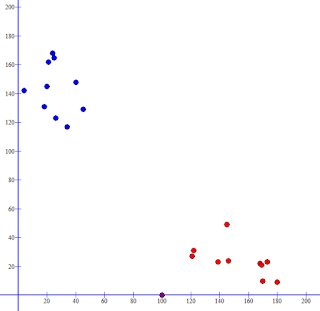## Sunday, December 13, 2015

### (k-)Nearest Neighbour(s) Classification

You want a computer to learn to assign objects into categories, such as the genre of a book. You happen to have a bunch of books with a known category. One of the simplest ways to make the computer assign an unknown book's category is to find the most similar book in the bunch to the unknown book and assume that the two books share the same category. For example, you want to find what genre "Harry Potter" is and find that it is most similar to a book you have called "The Hobbit" which is tagged as fantasy, so you conclude that "Happy Potter" is also fantasy. Of course this only makes sense if you have a big collection of reference books since there might not be any books which are similar otherwise, and the most similar book would be of a genre which is significantly different.

This is called the nearest neighbours classification algorithm, in particular the 1-nearest neighbour, because you only take into consideration the most similar book. Alternatively you can take the top 10 most similar books and use the most frequent genre among the 10 books. This would be called 10-nearest neighbours classification. In general it's called k-nearest neighbours classification.

This is a simple algorithm but its advantage is in its simplicity since it makes no assumptions about the data you give it. Whereas other machine learning algorithms assume that there is some simple pattern to decide which genre a book belongs to, the nearest neighbour classifier can discriminate between very complex patterns and will adapt to any data you train it with, provided that there is enough variety of data. The more complex the relationship between the books and the genre, the more variety of books you need to train it with.

The way it works is by first converting each book in your bunch into a bunch of lists of numbers called a vectors. Each vector would be a point in space (a vector of 2 numbers is a 2D point, of 3 numbers is a 3D point, and the rest are of high dimensional space). For example, in order to convert a book into a point, each number could be the number of times a particular word occurs. Create a vocabulary of words that matter, such as "wizard" and "gun", and then create a point consisting of the number of times each word occurs in the book. So if "Happy Potter" had "wizard" appearing 100 times and "gun" appearing 0 times, then it's 2D point would be (100, 0).

Next, compare the point version of the book in question to the point versions of every book in the bunch. Use some similarity measure to quantify how similar the points are. Similarity measures include Euclidean distance (normal distance between points) and Cosine similarity (difference in the angle of the points from the origin).Which is the most similar point to the purple one in the above diagram (the purple point is (100, 0) which represents "Harry Potter")? The purple point will be of the same colour as the closest point.

Of course comparing to every point is slow, which is a problem given that nearest neighbour classification requires a lot of points to compare to. There are nearest neighbour search algorithms but they are not very efficient when the points have a lot of dimensions (many numbers in the vector). In some cases it is enough to use approximate search algorithms that do not give exact nearest point, but will find a reasonably close point quickly. The paper "Scaling Distributional Similarity to Large Corpora" gives an overview of such algorithms for finding words that have similar meanings.

If you do not have the genres of the books but still want to categorize similar books together you can use a clustering algorithm such as k-means clustering into order to group books by similarity and then use nearest neighbour classification to associate the new book with the group of the nearest book.